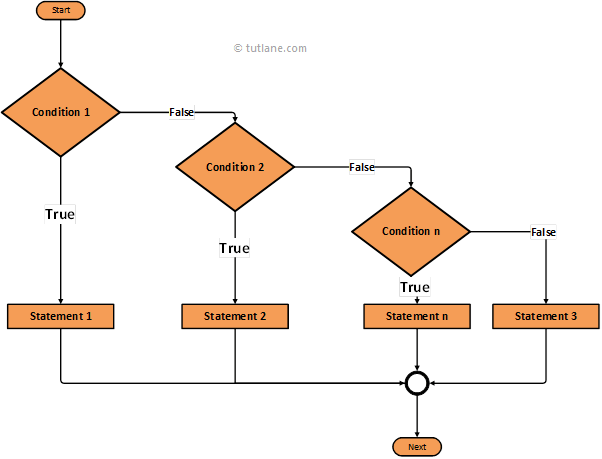# Swift If, Else If, Else Statement

In swift, if else-if else statement is used to add alternative set of multiple else-if and single else statements for if condition. In case if condition fails (FALSE) then alternatively check for another condition in case if it failed for all defined conditions it will execute else statements.

If we want to add multiple condition checks in a single program then by using swift if else-if else statement we can easily add multiple conditions. In if else-if else statement, we have a chance to add multiple else if statements but we are restricted to add only one if and else conditions in statement.

Now we will see the functionality of if else-if else statement in swift programming language using algorithm diagram.

## Swift If Else-if Else Statement Flow Diagram

Following is the swift if else-if else statement flow diagram to represent how the functionality of if else-if else statement will work in swift programming language.If you observe above swift if else-if else statement flow diagram first it will evaluates the first condition whether is it TRUE or FALSE. In case if first condition is TRUE then it will execute the code block within if statement. In case the given condition is FALSE then it will go for another condition and check for whether it’s TRUE or FALSE based on the status and it will execute required statements.

In case if all defined if and else-if conditions fails then the else condition statements will execute and reach the end of statement.

## Syntax of Swift If Else-If Else Statement

Following is the syntax of if else-if else statement in swift programming language.

if condition1

{

//Execute when it true

}

else if condition2

{

//Execute when other conditions fail and it's true

}

else if condition3

{

//Execute when other conditions fail and it's true

}

else

{

//Execute when all other conditions fail

}

Now we will see how to use if else-if else statement in swift programming language with examples.

## Swift If Else-if Else Statement Example

Following is the simple example using if else if else statement in swift to check the defined conditions before executing the expressions within the condition.

var num = 200

if num == 10 {

print("Given Number Equal to 10.")

}

else if num == 200 {

print("Given Number Equal to 200")

}

else {

print("Fail")

}

If you observe above example first we are check if condition whether the value of “num” equals to 10 or not. In case if it failed it will go to next else if condition and check the value of “num” equals to 200 or not even if that condition also failed then it will execute else condition by default.

When we execute above code in the swift playground we will get a result like as shown below.

Given Number Equal to 200

This is how we can use swift if else-if else statements in swift programming language to define multiple conditions based on our requirements.